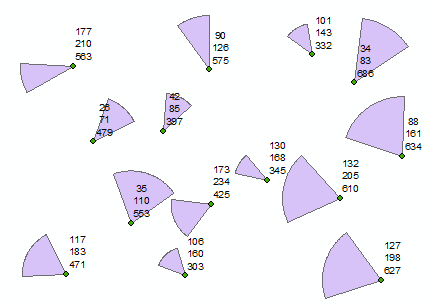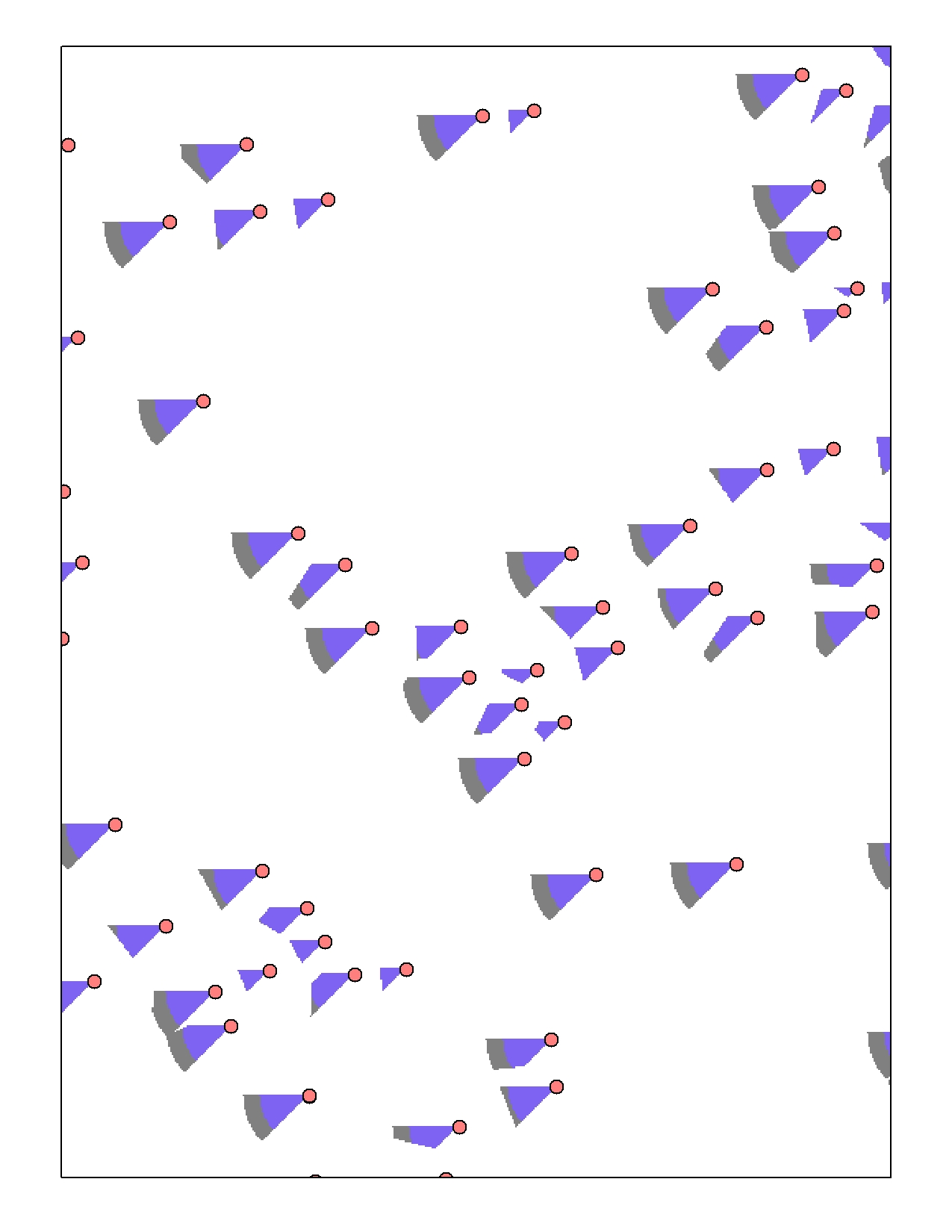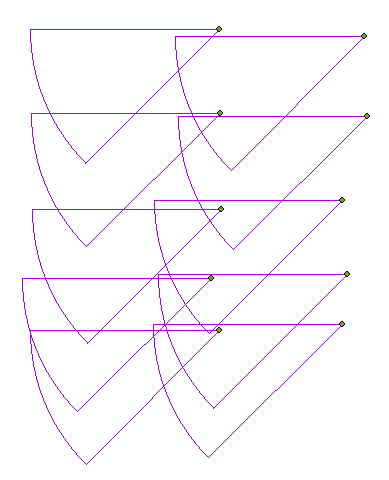# Oriented buffers

6701
28
02-07-2015 07:58 PMNew Contributor II

Hi there,

I am using arcview 9.3.1 and I need to create oriented buffers from points in a shapefile. The euclidean direction method almost works, but because it calculates to the nearest point, buffers associated with points within the maximum will be truncated. I need each point to have a complete buffer in a specific direction, regardless of overlap with other points.

Any ideas would be greatly appreciated.

Thanks,

Dave

Tags (3)
1 Solution

Accepted SolutionsbyEsri Esteemed Contributor

As promised...

The code below will create the circle sectors based on the point location and 3 additional columns:

• one for the start angle
• one for the end angle
• and the distance (size)

The angles are expressed arithmetically:In your case you will want to use start = 180° and end = 225°

I used random points, with random angles and random sizes and this was the result:This is the code I used:

```#-------------------------------------------------------------------------------
# Name:        createSectors.py
# Purpose:    create circle sectors for points
#
# Author:      Xander
#
# Created:    08-02-2015
#-------------------------------------------------------------------------------
import arcpy

def main():
fc_in = r"C:\Forum\CircleSectors\data.gdb\points2"
fc_out = r"C:\Forum\CircleSectors\data.gdb\sectors2"

fld_start = "StartAngle"
fld_end = "EndAngle"
fld_dist = "Length"

sr = arcpy.Describe(fc_in).spatialReference
arcpy.env.overwriteOutput = True

flds = ("SHAPE@", fld_start, fld_end, fld_dist)
lst_polygons = []
with arcpy.da.SearchCursor(fc_in, flds) as curs:
for row in curs:
pnt_g = row
start = row
end = row
length = row
circle = pnt_g.buffer(length)
pnt = pnt_g.firstPoint
arrPnts = arcpy.Array()
for bearing in range(int(start), int(end) + 1):
polygon = arcpy.Polygon(arrPnts, sr)
lst_polygons.append(polygon)

arcpy.CopyFeatures_management(lst_polygons, fc_out)

def createPointAtAngleWithBearing(pnt, angle, distance):
import math
dist_x, dist_y = (distance * math.cos(angle), distance * math.sin(angle))
return arcpy.Point(pnt.X + dist_x, pnt.Y + dist_y)

if __name__ == '__main__':
main()```

Kind regards, Xander

28 RepliesbyEsri Esteemed Contributor

Would the table to ellipse tool be of any help?

If not a picture to show what you are after would help.New Contributor II

Hi Xander,

Thanks for taking the time to help out. I'll try this tool out, but I need a different shape so it may not be the solution. I'll attach an image from the euclidean direction tool that will hopefully make this a bit clearer. Without getting into too much detail, I need to illustrate an area that may be a source for the point data. In the attached image, the transport direction ranges from 45-90 degrees, hence the cone shape (the transport vectors vary throughout the data set). My only issue is the truncation in the raster due to the close points. I.e, they need to overlap.

Another idea is to somehow generate 2 lines at specified azimuths from the points and then use them to intersect with a buffer around the point. This would allow for the overlap that the raster does not accommodate.

Pretty stumped right now, so I really appreciate your input and time.

DavebyMVP Honored Contributor

Here's how you can do this using arcpy and geometry objects:

```>>> radius = 200000 # buffer radius
>>> polys = []
>>> sr = arcpy.Describe("YOUR_POINT_LAYER_NAME_HERE").spatialReference
>>> with arcpy.da.SearchCursor("YOUR_POINT_LAYER_NAME_HERE","SHAPE@",'#',sr) as cursor:
...     for row in cursor:
...         pt1 = row.centroid
...         pt2 = arcpy.Point(row.centroid.X - radius*2, row.centroid.Y)
...         triangle = arcpy.Polygon(arcpy.Array([pt1,pt2,pt3]),sr)
...         wedge = triangle.intersect(buffer,4)
...         polys.append(wedge)
...
>>> arcpy.CopyFeatures_management(polys,'in_memory\wedges')
<Result 'in_memory\\wedges'>```It helps that all wedges are the same shape, so the triangle shape used to clip (intersect) the buffer is hard-coded. You could fairly easily alter the script to respond to attribute data.

Sorry for swooping in on your fun, XanderbyEsri Esteemed Contributor

LOL, it's actually a very nice a simple way of getting the result... So no harm done, thanks for contributing this solution, Darren Wiens‌ and yes I think this would answer the question.

I will however start with a more generic solution that allows for setting the start angle, end angle and distance for each sector and will share it later on.byEsri Esteemed Contributor

As promised...

The code below will create the circle sectors based on the point location and 3 additional columns:

• one for the start angle
• one for the end angle
• and the distance (size)

The angles are expressed arithmetically:In your case you will want to use start = 180° and end = 225°

I used random points, with random angles and random sizes and this was the result:This is the code I used:

```#-------------------------------------------------------------------------------
# Name:        createSectors.py
# Purpose:    create circle sectors for points
#
# Author:      Xander
#
# Created:    08-02-2015
#-------------------------------------------------------------------------------
import arcpy

def main():
fc_in = r"C:\Forum\CircleSectors\data.gdb\points2"
fc_out = r"C:\Forum\CircleSectors\data.gdb\sectors2"

fld_start = "StartAngle"
fld_end = "EndAngle"
fld_dist = "Length"

sr = arcpy.Describe(fc_in).spatialReference
arcpy.env.overwriteOutput = True

flds = ("SHAPE@", fld_start, fld_end, fld_dist)
lst_polygons = []
with arcpy.da.SearchCursor(fc_in, flds) as curs:
for row in curs:
pnt_g = row
start = row
end = row
length = row
circle = pnt_g.buffer(length)
pnt = pnt_g.firstPoint
arrPnts = arcpy.Array()
for bearing in range(int(start), int(end) + 1):
polygon = arcpy.Polygon(arrPnts, sr)
lst_polygons.append(polygon)

arcpy.CopyFeatures_management(lst_polygons, fc_out)

def createPointAtAngleWithBearing(pnt, angle, distance):
import math
dist_x, dist_y = (distance * math.cos(angle), distance * math.sin(angle))
return arcpy.Point(pnt.X + dist_x, pnt.Y + dist_y)

if __name__ == '__main__':
main()```

Kind regards, XanderNew Contributor II

Thank you both. You guys are awesome. Seriously. Xander, will Darren see this? not sure how to tag him.

I think the variable sizes and angles will work perfectly. But.. and this is a big one... I am using Arcview 9.3.1, so as far as I can tell, I can't use arcpy. I will try to get access to a machine with a higher version.

I'm not familiar with the commands for arcpy, although it appears it's time to learn. With the inputs referenced from the shapefile, you've bang on solved my problem. Don't even want to think about how many hours I could have saved with other problems.

So.. I'm not sure if this should be under a new thread, but the next step is to determine the percentage of another layer within each of these polygons. Or, more accurately, the types and proportion of each bedrock unit within each polygon. I've just started researching this, but I thought I should ask you guys before I spend too many hours..

I wish I could thank you guys more for the help. It's much appreciated.byEsri Esteemed Contributor

You are right, it will not work on 9.3.1. And since I am making use of the data access module, You will need to have access to ArcGIS 10.1 SP1 or higher. It would be good to upgrade, since each new version of ArcGIS provides some new goodies. The current version is 10.3.

I don't have access to 9.3.x, so it would be difficult to rewrite it for this version. I could rewrite it for 10.0 and 10.1, but if you are going to upgrade, it's best to pick a higher version. The alternative is to upload your featureclass and I can run it for you and attach the result to this thread.

To learn arcpy (and python) there are a lot of threads where this has been answered and useful links to resources have been provided, like this one: Seeking advice on how to go about learning Python

For getting info on the bedrock units per polygon, you could use a union and analyze the attributes. If you have any problems with that, you can start a new thread (this will attract the attention of more people and will result in more response).

Kind regards, XanderNew Contributor II

Another quick question. Is it possible to have the point ID field recorded as an attribute in the resulting polygon?

Thanks again!byEsri Esteemed Contributor

Yes, it is, but will require some rewriting of the code. I will get back on this in a moment...